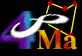##Mathematics

• * Flash Cards for Kids: A fun online interface for learning basic addition, subtraction, multiplication and division. Students can set the level of complexity and whether or not to keep score.
• MegaMath: Online tutorials geared towards K12 students for a variety of branches of mathematics from algebra to topology (knot theory).
• * S.O.S. MATHematics: Online units to explain topics in algebra, trigonometry, calculus, matrices and complex variables.

### More Math..

Reference:
• Calculators Online: A comprehensive listing of online applets and free programs that can be used to make conversions and a varity of calculations in engineering, mathematics and other areas of science.

Dr. Math: Staffed by students at Swarthmore College.

## Careers in Mathematics

American Mathematical Society: e-Math Careers Page.

## WWW Virtual Libraries

Virtual Library for Mathematics

## USENET Groups

sci.math. Also - sci.math.num-analysis, and stat.math - statistics.